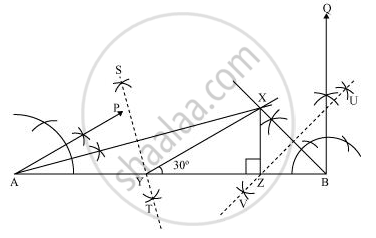# Construct a triangle XYZ in which ∠Y = 30°, ∠Z = 90° and XY + YZ + ZX = 11 cm. - Mathematics

Construct a triangle XYZ in which ∠Y = 30°, ∠Z = 90° and XY + YZ + ZX = 11 cm.

#### Solution

The below given steps will be followed to construct the required triangle.

Step I:- Draw a line segment AB of 11 cm.

(As XY + YZ + ZX = 11 cm)

Step II:- Construct an angle, ∠PAB, of 30° at point A and an angle, ∠QBA, of 90° at point B.

Step III:- Bisect ∠PAB and ∠QBA. Let these bisectors intersect each other at point X.

Step IV:- Draw perpendicular bisector ST of AX and UV of BX.

Step V:- Let ST intersect AB at Y and UV intersect AB at Z.

Join XY, XZ.

ΔXYZ is the required triangle.Concept: Some Constructions of Triangles
Is there an error in this question or solution?

#### APPEARS IN

NCERT Class 9 Maths
Chapter 11 Constructions
Exercise 11.2 | Q 4 | Page 195